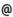## "Babes-Bolyai" University of Cluj-Napoca Faculty of Mathematics and Computer Science

 Finite element and boundary element method
 Code Semes-ter Hours: C+S+L Credits Type Section ME281 1 2+2+0 8 compulsory Matematică Aplicată ME281 1 2+2+0 9 compulsory Modele matematice în Mecanică şi Astronomie
 Teaching Staff in Charge
 Prof. TRIF Damian, Ph.D., dtrifmath.ubbcluj.ro
 Aims The course aims to provide a thorough understanding of mathematical principles, numerical and implementational aspects of the method. Extensive computer exercises shall be regarded as important tools for reaching the major goals.
 Content 1. Numerical methods for partial differential equations 2. Finite element method introduction (examples, variational formulation, error estimation, least squares-finite element method) 3. 2D model problems, Poisson equation, computer implementation 4. Abstract formulation 5. Finite element spaces 6. Piecewise linear function - approximation theory 7. Triangulation generation 8. Direct and iterative solvers for large and sparse linear systems 9. Parabolic problems (semi- and complete discretization, discontinuous Galetkin method) 10. Hyperbolic problems (standard Galerkin method, artificial diffusioon, upstream diffusion) 11. Hyperbolic problems - discontinuous Galerkin method 12. Nonlinear problems 13. Boundary element method 14. Finite volume method, particle method
 References 1. GHEORGHIU C. I., A constructive introduction in finite element method, Quo Vadis, 1999 2. JIANG B. N., The least-square finite element method, Springer-Verlag, 1998 3. JOHNSON C., Numerical solution of partial differential equations by the finite element method, Cambridge Univ. Press, 1987 4. PETRILA T., GHEORGHIU C. I., Metode element finit si aplicatii, Ed. Academiei, 1986 5. PETRILA T., TRIF D., Metode numerice si computationale in dinamica fluidelor, Ed. Digital Data, Cluj, 2002 6. QUARTERONI A., VALI A., Numerical approximation of partial differential equations, Springer-Verlag, 1994
 Assessment Midterm exam (theory + matlab code) 30% Final written exam (theory) 60%